Formula Quiz
Give best answer.
Your name *
Your answer
The n in summation notation is the *
1 point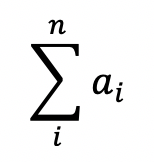This formula is used to *
1 point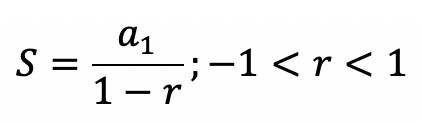Summation notation is also known by the greek letter for ______ which is called ______ *
1 pointThis formula is used to *
1 point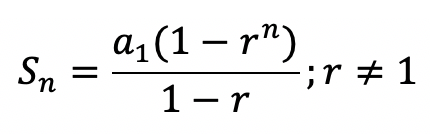5i represents the *
1 point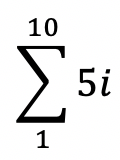Give the first and last terms of this summation *
1 pointThis formula is used to *
1 point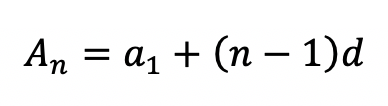The i in summation notation is the *
1 pointThis formula is used to *
1 point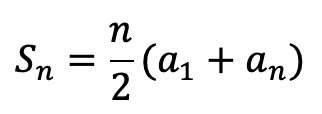This formula is used to *
1 point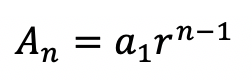Never submit passwords through Google Forms.
This content is neither created nor endorsed by Google. Report Abuse - Terms of Service